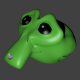Green Noob -3 years ago 124
C Question

# Deleting an array element in C

I wrote the following program to delete an array element entered by the user.

``````#include<stdio.h>
#include<conio.h>

void main()
{
int j,i,a,n,key,l;
clrscr();
printf("Enter the number of elements:");
scanf("%d",&n);
printf("\nEnter the elements:\n");
for(i=0;i<n;i++)
scanf("%d",&a[i]);
printf("\nEnter the element to delete:");
scanf("%d",&key);
l=n;  //Length of the array
for(i=0;i<l;i++)
{
if(a[i]==key)
{
for(j=i;j<l;j++)
a[j]=a[j+1];
l--;    //Decreasing the length of the array
}
}

printf("\nThe new array is \n");
for(i=0;i<l;i++)
printf("%d ",a[i]);
getch();
}
``````

It works fine for most inputs but when the input is something like: 1 2 2 3 5 (here 2 repeats consecutively) and the element to be deleted is 2, the output is 1 2 3 5.

How can I modify the program such that all instances of the entered element is removed?Shadow
Answer Source

After l-- put i-- too as shown below

``````if(a[i]==key)
{
for(j=i;j<l;j++)
a[j]=a[j+1];
l--;    //Decreasing the length of the array
i--;    //check again from same index i
}
``````
Recommended from our users: Dynamic Network Monitoring from WhatsUp Gold from IPSwitch. Free Download
Latest added